MORE IN Signals & Systems
Total marks: --
Total time: --
INSTRUCTIONS
(1) Assume appropriate data and state your reasons
(2) Marks are given to the right of every question
(3) Draw neat diagrams wherever necessary

1 (a) Sketch the even and odd part of the signal shown in Fig. Q1 (a)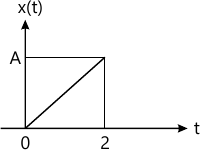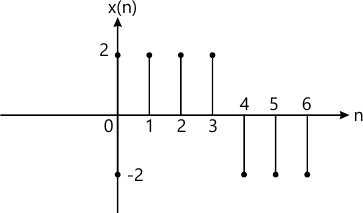6 M
1 (b) Check whether the following signals is periodic or not and if periodic find its fundamental period.
i) x(n)=cos(20?n)+sin(50?n)
ii) x(t)=[cos(2?n)]2
6 M
1 (c) Let x(t) and y(t) as show in Fig Q1(c). Sketch (i) x(t)y(t-1) (ii) x(t)y(-t-1)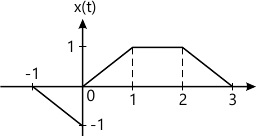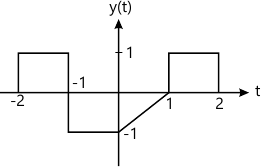8 M

2 (a) Determine the convolution sum of the given sequences
x(n)={1, -2, 3, -3} and h(n)={-2, 2, -2}
4 M
2 (b) Perform the convolution of the following sequences:
x1(t)=e-at: 0?t?T
x2(t)=1: 0?t?2T
10 M
2 (c) An LTI system is characterized by an impulse response, $h(n)=\eft ( \dfrac {1}{2} \right )^n u(n.)$ Find the response of the system for the input $x(n)= \left ( \dfrac {1}{4} \right )^n u(n).$
6 M

3 (a) Determine the following LTI system characterized by impulse response is memory, casual and stable.
i) h(n)=2u(n)-2u(n-2)
ii) h(n)=(0.99)nu(n+6).
6 M
3 (b) Find the natural response of the system described by a differential equation $\dfrac {d^2 y(t)}{dt^2}+ 2 \dfrac {dy(t)}{dt}+2y(t)=2x(t), \ with \ y(0)=1, \ and \ \left.\begin{matrix} \dfrac {dy(t)} {dt}\end{matrix}\right|_{t=n}=0$
6 M
3 (c) Find the difference equation description for the system shown in Fig Q3(c).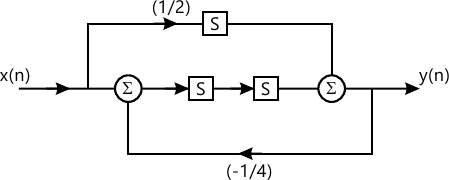4 M
3 (d) By converting the differential equation to integral equation draw the direct form-I and direct form-II implementation for the system as $\dfrac {d^3 y(t)}{dt^3}+ \dfrac {d^2 y(t)}{dt^2}+ 2 \dfrac {dy(t)}{dt}= x(t)+ 6 \dfrac{d^2 x(t)}{dt^2}$
4 M

4 (a) State and prove the following properties of DTES: i) Modulation ii) Parseval's theorem.
10 M
4 (b) Find the Fourier series coefficient of the signal x(t) shown in Fig Q4(b) and also draw its spectra.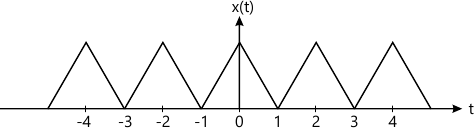10 M

5 (a) Find the DTFT of the following signals:
i) x(n)=a|n|; |a|<1
ii) x(n)=2n u(-n)
8 M
5 (b) Determine the signal x(n) if its DTFT is as shown in Fig. Q5(b).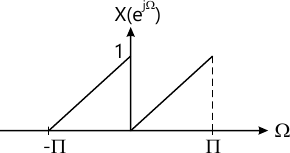6 M
5 (c) Compute the Fourier transform of the signal $x(t)= \left\{\begin{matrix} 1+\cos \pi t&;&|t|\le 1 \\0 &; &|t|>1 \end{matrix}\right.$
6 M

6 (a) Find the frequency response of the system describe by the impulse response h(t)=?(t)-2e-2t u(t) and also draw its magnitude and phase spectra.
8 M
6 (b) Obtain the Fourier transform representation for the periodic signal x(t)=sin w0t and draw the magnitude and phase.
7 M
6 (c) A signal x(t)=cos(20?t)+1/4 cos(30?t) is sampled with sampling period ?s. Find the Nyquist rate.
5 M

7 (a) What is region of convergence (ROC)? Mention its properties.
6 M
7 (b) Determine the z-transform and ROC of the sequence $x(n)=r_1^n u(n)+ r^n_2 u(-n)$
7 M
7 (c) Determine the inverse z-transform of the function, $x(z)= \dfrac {1+z^{-1}}{1-z^{-1}+0.5z^{-2}}$ using partial fraction expansion.
7 M

8 (a) An LTI system is described by the equation
y(n)=x(n)+0.8 x(n-1)+0.8x(n-2)-0.49y(n-2)
6 M
8 (b) Determine the transfer function H(z) of the system and also sketch the poles and zeros.
8 M
8 (c) Determine whether the system described by the equation y(n)=x(n)+by(n-1) is causal and stable where |b|<1. Find the unilateral z-transform for the sequence y(n)=x(n-2), where x(n)=?n.
6 M

More question papers from Signals & Systems